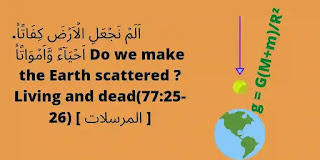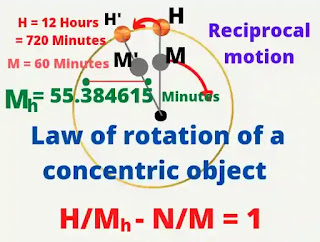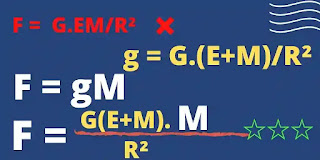# Religion according to scienceReligion according to science
Religion according to science : I'm basically talking about astronomy here. Truth be told, this astronomy has been described the most in the Qur'an, the world's best religious book on science. On the other hand, apart from medical science, this astronomy subject has been the most researched in the world. I will mainly talk about the tested science here, I will talk about physics and especially on mathematics. In order to reveal the fact that Allah, the Great Creator, has made His creation more mathematical in a higher way. I will now discuss only the earth, the moon and the sun. I will discuss the planets later. Now let's talk about this religion according to science.Speed ​​in the same direction
According to all the scriptures of the world, our Earth is fixed in the universe.
The Qur'an declares :      وَمِنۡ اٰيٰتِهٖۤ اَنۡ  تَقُوۡمَ السَّمَآءُ وَالۡاَرۡضُ بِاَمۡرِهٖ ؕ
"  And among His signs that heaven and earth stand by His command. "    [ 30 : 25 ]
The scriptures do not say how our world is stable. In my opinion, the stability of the earth in this universe is a great sign of the Creator. The truth is that we have no evidence of how the earth is fixed in this universe.

## Some important astronomical fact :

1. The earth is round and stationary in the universe.
2.  The earth completes one orbit on its own axis in about

86164.0996 seconds Or 23 hours 56 minutes 4.100 seconds ( Upcoming values ​​up to three decimals ).Diurnal motion

3. The rotation period of the celestial-pole is anticlockwise direction.  It would take about 25,793 years to complete one rotation. Basically we see the precession of equinoxes as a result. We see that the first point of Aries ( and therefore first point of Libra also) moves slowly backward ( opposite direction of the sun's annual motion among the stars) along the ecliptic and it would take 26,000 years. The celestial-pole describes a circle ( in the anticlockwise direction ) about the people of the ecliptic, always remaining at a constant distance ш ( about 23°27' ).

4. The celestial pole sometimes comes towards the pole of ecliptic and sometimes away from this, this nodding as it were of the celestial pole is called Nutation. And this cycle is completed after about 18.5966 years. These motions of the earth are basically noticeable.

### New definition of Ecliptic :

The moon and the sun orbit our earth according to a certain plane is called the Ecliptic. The ecliptic is an imaginary circle in which the moon and sun orbit the earth. It is inclined to the plane of the equator at an angle of about 23.44°. The moon is inclined to the plane of the Ecliptic at an angle of about 5.14° and the sun is inclined to the plane of the Ecliptic at an angle of about 1.25° .

#### Revolution period of the moon's Nodes  :

The points of intersection of the moon's apparent orbit, with the ecliptic are called moon's Nodes. These are not fixed points but have a retrograde motion along the ecliptic at the rete of about 19° in a year completing a revolution period in about 18.60 years.  This is the revolution period of the moon's Nodes.

#### Revolution period of the sun's Nodes  :

The points of intersection of the sun's apparent orbit, with the ecliptic are called sun's Nodes. These are not fixed points but have a retrograde motion along the ecliptic at the rete of about 31.61" in a year completing a revolution period in about 41,000 years.  This is the revolution period of the sun's Nodes. The two lunar Nodes change the position of each other in   ( 18.6  ÷ 2 ) = 9.3 years. It's result we can see moon's orbit inclined changes to the plane of the equator at an angle of about 18.30° to 28.58°. And The two solar Nodes change the position of each other in   ( 41,000 ÷ 2 ) = 20,500 years. It's result we can see sun's orbit inclined changes to the plane of the equator at an angle of about 22.1° to 24.5°. This results in massive changes in the monsoon winds every 20,000 years. It is believed that this change in the monsoon winds turned the desert into a reservoir.

#### To determine the period of the moon and the sun, orbiting the earth :

The earth is fixed point in this universe and the stars are also remain in fixed points  So the sidereal day is the actual orbital period of the moon and the sun

Revolution period of the moon is 27.32166 days ( 2360591.5 seconds ) to complete one orbit around the earth.

Revolution period of the sun is 365.256363 days       ( 31558149.5 seconds ) to complete one orbit around the earth.

#### Lunar day :

The Lunar day is the earth rotation period relative to the Moon. Here,

M = 2360591.5 seconds  or  27d 7hr 43min 11.5sec

E = 86164.0996 seconds Or  23hr 56mi 4.0996sec.

They are concentric and moving in the same direction. Keep, Lunar day  Em  , So

Em = M×E/(M - E) sec.

= 89428.33 seconds

= 24hr 50min 28.33sec.

So Lunar day is 24 hours 50 minutes and 28.33 sec.

#### Solar day :

The Solar day is the earth rotation period relative to the sun.   Here,

S = 31558149.5 seconds

E = 86164.0996 seconds        They are concentric and moving in the same direction. Keep, Solar day is Es .                                  so, Es = S×E/(S - E)

= 86400.000 Seconds

So the Solar day is 24 hours only.

#### Arabic lunar month :

The orbit of the moon from the earth, relative to the sun, is called the Arabic lunar month or Synodic month or Lunar month. Keep, revolution period of the moon relative to the sun Ms  .

S = 31558149.5 seconds

M = 2360591.5 seconds

They are concentric and moving in the same direction. So,

Ms = S.M/(S - M)

We get from this equation

Ms = 2551442.811 sec.               ~ 2551443 seconds.         = 29d 12hr 44min 03sec Or 29.53059 days

#### So, one secular Arabic lunar month equals 29.53059 days

Now think about how much the world of creation agrees with mathematics and where religion is wrong.

## Now I will discuss retrograde motion :Retrograde motion

We know that the first point of Aries ( and therefore first point of Libra also ) moves slowly backward ( i.e. ,in the direction opposite to that of the sun's  annual motion among the stars ) along the ecliptic. Keep, revolution period of the first point of Aries Or rotation period of the Earth's Pole P .

Revolution period of the Sun is 31558149.5 sec.

The sun moves relative to the First point of Aries Sp .

We know,

Sp = 31556925.9747 sec.

The Sun and  First point of Aries are concentric and their motions are opposite to each other. So,

P = S×Sp/(S - Sp)

= 813941638698.0 sec.

= 25792.8 years

So,  the First point of Aries moves slowly backward along the ecliptic and it would take about 25,792.8 years to complete one rotation. We call the motion precession of equinoxes. The precessional motion is about ( 360×3600 ÷ 25792.8 ) = 50.24658" per year. This is due to the rotation of the Earth's polar axis.

#### TROPICAL DAY :

Rotation period of the Earth relative to the First point of Aries. We know,
revolution period of the first point of Aries Or rotation period of the Earth's Pole P .
P = S×Sp/(S - Sp)

= 813941638698.0 sec. And sidereal day Or rotation period of the Earth E

E = P.Ep/(P - Ep)

= 86164.09962 seconds

~ 86164.0996 seconds

Their motions are opposite to each other.

Keep Tropical Day Ep

so, Ep = P×E/(P + E)

We get from this equation

Ep = 86164.09050 sec.

= 23hr 56mi 4.0905 sec.

So the Earth rotates own his axis in 23hr 56mi 4.0905 seconds relative to the First point  of ries. We can call it a topical day. So,

#### there are four types of days :

1. Sidereal day
2. Tropical day
3. Solar day
4. Lunar day

1. Sidereal day or rotation period of the earth :  It is the true rotation period of the Earth.

E = 86164.0996 seconds

2. Tropical day : Rotation period of the Earth relative to the First point of Aries.

Ep = 86164.0905 seconds

3. Solar day : Rotation period of the Earth relative to the sun.

Es = 86400 seconds

= 24 Hour

4. Lunar day : Rotation period of the Earth relative to the Moon.

Em = 89428.3386 seconds

= 24hr 50min 28.34 sec.

#### Four kinds of  year :

1. Sidereal year
2. Tropical year
3. Anomalistic year
4. Nodical year

1. Sidereal year - It is the interval between two successive passages of the sun through the same point relative to fixed stars 🌟. It is the true period of the sun's revolution round the Earth.
Keep sidereal year is S
S = 365.256363d
= 365d 6hr 9min 9.5sec
=31558149.5 seconds

2. Tropical year ( Sp ) - It is the interval between two successive passages of the sun through the First point of Aries that's mean the sun moves relative to the Earth's precession.

P = 813941638698.0 sec.

S = 31558149.5 seconds

Their motions are opposite to each other.

Sp = P×S/(P+S)

= 31556925.9747 sec.

= 365d 5hr 48min 45.9747 seconds

= 365.2421987 days

So Tropical year is about 365.2422 mean solar day.

3. Anomalistic year - It is the interval between two successive passages of the sun through the apogee. Animalistic year is about 365.2596 mean solar days. 4. Nodical year - Revolution period of the sun relative to the Nodes   ( Sn) is called Nodical year.

S = 365.256363 days

N = 6792.2755 days

Their motions are opposite to each other.

The Sun moves relative to the Node --- Sn

So,   Sn = N×S/(N+S)

We get from this equation

Sn = 346.6169 days

So the Nodical year is in about 346.6169 days.

#### Five kinds of month :

1. Sidereal month
2. Tropical month
3. Anomalistic month
4. Nodical month
5. Synodic month

1. Sidereal month - It is the interval between two successive passages of the moon through the same point relative to fixed stars 🌟. It is the true period of the moon's revolution round the Earth. One sidereal month is made in about 27.32166 days.
M = 2360591.5 seconds
2. Tropical month (Mp) - It is the interval between two successive passages of the Moon through the First point of Aries that's mean the sun moves relative to the Earth's precession.

P = 813941638698.0 sec.

M = 2360591.5 seconds

Their motions are opposite to each other. So,

Mp = P×M/(P+M)

= 2360584.65 seconds

=  27.3218 days

3. Anomalistic month - It is the interval between two successive passages of the Moon through the apogee. Animalistic manth is about 27.5546 mean solar days.

4. Nodical month - Revolution period of the Moon relative to the Nodes (Mn) is called Nodical month.

S = 27.3217 days

N = 6792.2755 days

Their motions are opposite to each other.

The Moon moves relative to the Node --- Mn

So,   Mn = N×M/(N+M)

We get from this equation

Sn = 27.2122 days

So the Nodical month is in about 27.2122 days.

5. Synodic month : Rotation period of the moon relative to the sun is called the synodic month.

The moon round the Earth once in 2360591.5 sec.

The sun round the Earth once in 31558149.5 sec.

S = 31558149.5 seconds

M = 2360591.5 seconds

Keep, the moon round the Earth, relative to the sun in Ms seconds.

Ms = S.M/(S-M)
= 2551442.8 seconds
= 29d 12hr 44min 03sec
= 29.53059 days

So, the mean length of the Synodic month is 29 days 12 hours 44 minutes 03 seconds

#### Repetition of phases of the moon :

After 235 Arabic lunar months, the moon and sun return to almost the same place in their orbits. And we call this cycle, the metonic cycle.

235 Synodic months

= 235 × 29.53059 days

= 6939.69 days

19 sidereal years

= 19 × 365.2564 days

= 6939.87 days

254 sidereal months is equal to

254 × 27.3217 days

= 6939.71 days

~ 6940 days ~ 19 tropical years.

It fallows that after a period of 6940 days, the sun and the moon will occupy almost exactly the same position relative to the start and phases of the moon repeate ( occur ) again. This period is called Metonic cycle. The repetition of eclipses organized in exactly the same way.

#### repetition of eclipses :

223 After the Arabic lunar month, Nodes of the sun and the moon return to the same place. This results in a recurrence of the eclipse.
223 Synodic months
= 223 × 29.5306 days
= 6585.32 days
19 Nodical years
= 19 × 346.6169 days
= 6585.72 days
242 Nodical months
= 242 × 27.2122 days
= 6585.35 days
~ 6585 days
~ 18.0291 years
It is follows that after a period of 18 years,  the moon and the sun will occupy almost exactly the same position relative to the Nodes and the eclipses repeat them selves. This period is called the Chaldean Saros.

#### Relation between the tropical year and Arabic year :

The Arabic year depends on the lunar month, one Arabic year is equal to twelve lunar months.
One Arabic year
= 12 × 29.5306 days
= 354.3672 days
One Tropical year
= 365.2422 year
Difference between the tropical year and the arabic year is 10.8750 days.
So the Arabic year will occur at exactly the same time of the tropical year after ( 365.2422 ÷ 10.875 )
= 33.5855 years.

#### The magic of modern gravitational science :

اَلَمۡ نَجۡعَلِ الۡاَرۡضَ كِفَاتًاۙ.
اَحۡيَآءً وَّاَمۡوَاتًاۙ
Do we make the Earth scattered ? Living and dead
(77:25-26) [ المرسلات ]
Every object in this universe is floating in motion and every two objects attract each other with a force. The central stationary object and orbiting object attract each other with a force which is directly proportional to the product of the of sum of their masses and mass of the orbiting object, and inversely proportional to the square of the radius and it acts along the line joining the masses.
Every object in this universe is floating in motion and every two objects attract each other with a force. The central stationary object and orbiting object attract each other with a force which is directly proportional to the product of the of sum of their masses and mass of the orbiting object, and inversely proportional to the square of the radius and it acts along the line joining the masses.Modern gravitational science
New law of acceleration :

#### g = G. ( E + m )/R²

Here, the gravitational acceleration of a stationary object is  g
The universal gravitational constant is G
Mass of the stationary object  E
The small mass of the falling object is  m
The distance between the centre of the stationary object and the falling object is  R
So, the new law of gravitation is  " Any two particles in this universe attract each other with a force, As a result the moving object moves towards the stationary object with a balanced acceleration. This acceleration is directly proportional the sum of their masses and inversely proportional to the square of the distance between them. In the free state, that acceleration is proportional to their masses."
The fact is that the new law of gravitation is just a transformation of Newton's second law. So,
F = g.m
g = G.( E + m )/R²
We get from these two equations
F = G. ( E + m )/R².m
= G.(E +m)m/R²
That's mean,  Every object in this universe is floating in motion and every two objects attract each other with a force. The central stationary object and orbiting object attract each other with a force which is directly proportional to the product of the of sum of their masses and mass of the orbiting object, and inversely proportional to the square of the radius and it acts along the line joining the masses.
This is the perfect law of gravitation.
According to my formula, I have learned the value of G from the previohus POST2 and got the actual value of g from POST3. So, now we can determine the actual mass of the Earth.
Keep, mass of the earth M and the mass of another object is m ( 1 quintal = 100kg Or 10⁵ grams). We get from mass relation formula 》
M+m = gR²/G
M+ 10⁵ grams  = 6.017359559 × 10²⁷grams  Or
M = ( 6.017359559 × 10²⁷ - 10⁵ ) grams Or,
M = 6.017359559 × 10²⁷ g. The mass of 10⁵ grams of matter is very small compared to the mass of our Earth ( 6.0 × 10²⁷ grams) so it is ignored.
Here, Mass of the Earth = 6.017359559×10²⁷ grams
m = 10⁵ grams
G = ( 6.643385875 ÷ 10⁸ ) cm³/g.s²  So here,
R = 6371km = 6.371 × 10⁸ cm
g = G.(M+m)/R²
= 984.873 cm/s² . It is actual gravitational acceleration of the Earth. The force of attraction between the earth and that object is F.
Now let's see some more mathematical similarities.
F = g.m
= 984.873 × 10⁵ g.cm/s²
F = 98487300 dyne.
F = G.(M+m).m/R²

We will calculate from this equation

F = 984873 g.cm/s²  Or

## F = 98487300 dyne.

The force of attraction between the earth and that object is  98487300 dyne.